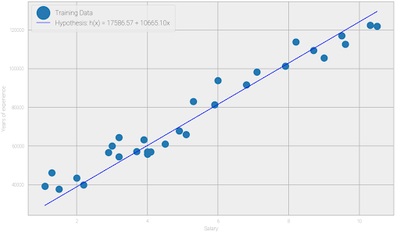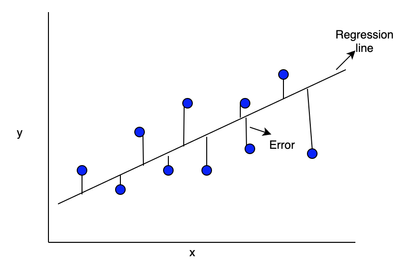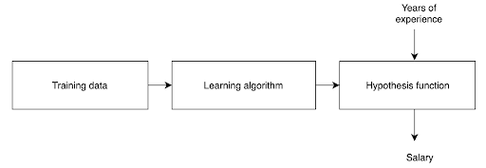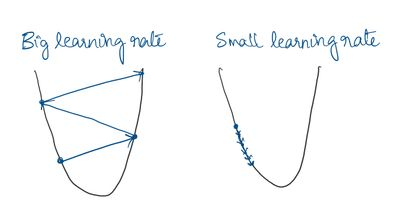Community Articles
Find and share helpful community-sourced technical articles
Announcements
Alert: Welcome to the Unified Cloudera Community. Former HCC members be sure to read and learn how to activate your account here.

## Understanding Linear Regression

Labels (1)
• ### Cloudera Data Science Workbench (CDSW)

srinijaCloudera Employee

Introduction

In this article we’re going to look at linear regression, which is a technique for estimating linear relationships between various features and a continuous target variable. Linear regression provides the ability to measure a correlation between your input data and a response variable. We’ll illustrate this concept with a simple example. Suppose you have collected data for employees’ years of experience and their corresponding salaries. To understand the distribution of this data we can generate a plot as shown in Fig 1. You can see that there’s a linear relationship between years of experience (x) and salary (y). This correlation allows us to build a linear regression model that outputs a salary given years of experience.Fig1 : Points plotted using training examples (years of experience, salary)

Let’s look at the internals of a linear regression algorithm. The  algorithm will fit a line in the form of y = β0 + β1x to your data. Here we call x the independent variable or input variable and y is called the dependent variable or output variable, β1 represents the slope of the line and β0 represents the intercept of the line. The goal of the algorithm is to find a line that best fits a set of points to minimize the error where the error is the distance from the line to the points.Fig 2: Describes the error which is the distance between actual value and the estimated regression line.

The line moves according to the parameter values (β0,β1). Similarly, you can perform multiple linear regressions, using multiple input variables, to improve the general applicability of the learning algorithm.

Linear regression algorithmFig3 : Showcasing Linear regression model using input variable as Years of Experience and Salary

Hypothesis function refers to the equation h(x) = β0 + β1x which maps input x (years of experience) to target y (salary).

Cost function: The cost function determines how to fit the best line to the data. For hypothesis function h(x) =  β0 + β1x choose β0 and β1 so that h(x) is close to y for training examples (x,y). This represents the optimization problem to solve.

Ordinary Least Square method: The regression model uses ordinary least squares methodology which is a summation of square of difference between actual value(y) and the predicted value(y')  which can be denoted as ∑[(y - y')]² for all training examples in the dataset  to find the best possible value of the regression coefficients β0 and β1

Optimization algorithm

Gradient descent is one of the methods that can be used to reduce the error, which helps by taking steps in the direction of a negative gradient. Gradient descent is an optimization algorithm that tweaks its parameters iteratively. In machine learning, gradient descent is used to update parameters in a model. Let us relate gradient descent with a real-life analogy for better understanding. Think of a valley you would like to descend, you first will take a step and look for the slope of the valley, whether it goes up or down. Once you are sure of the downward slope you will follow that and repeat the step again and again until you have descended completely (or reached the minima).Gradient descent does exactly the same. The algorithm makes use of learning rate parameter to take steps. Choosing the learning rate is an important step as big learning rate can skip the minima and very small learning rate can take infinite time to reach the minima.Fig 4: Very big learning rate can skip the minima and very small learning rate might take infinite steps to reach minima

Evaluating model performance

Once the model is built, the metrics used to determine model fit can have different values based on the type of data. Let’s consider a real life example seen in Fig1 where we have details of employee years of experience and salary and we want to find the correlation between them. Once the model is built we calculate R squared score as a metric of performance. If the R Squared score is 0.92 it means that 92% of the variability is covered by the model which is good because the high variability covered tells us that the model is very generalized. Similarly, the following are some metrics you can use to evaluate your regression model:

R Square (Coefficient of Determination): This metric describes the percentage of variance explained by the model. It ranges between 0 and 1. The value depends on the quality of the dataset. Generally higher R²  is desirable because it means model is more generalized.

Adjusted R²:  R square assumes that every single variable explains the variation in the dependent variable. The adjusted R square tells you the percentage of variation explained by the independent variables that actually affect the dependent variable. A model that includes several predictors will return higher R square values and may seem to be a better fit. However, this result is due to it including more terms.

The adjusted R-squared compensates for the addition of variables and only increases if the new predictor enhances the model above what would be obtained by probability. Conversely, it will decrease when a predictor improves the model less than what is predicted by chance.

Mean Squared Error (MSE): The average squared difference between the estimated values and the actual value. The MSE is a measure of the quality of an estimator, lower MSE values are desirable.

Mean Absolute Error (MAE): A measure of difference between two continuous variables. It is robust against the effect of outliers. Again, the lower MAE value the better.

Root Mean Square Error (RMSE): Interpreted as how far, on average, the residuals are from zero. It nullifies the squared effect of MSE by square root and provides the result in original units as data. Again, the lower the better.

Pseudo code for building Linear Regression model using scikit-learn

```# Import all the necessary libraries
#import your dataset in the place of example.csv
#specify your input and output variables based on your data, varies on different datasets
X= dataset.iloc[:,[2,3]].values
y= dataset.iloc[:,4].values
#Split the data into training and testing set using train_test_split
X_train,X_test,y_train,y_test=train_test_split(X,y, test_size=0.2,random_state=42)
#Perform feature scaling to transform data to fit into the model
X_train=sc_X.fit_transform(X_train)
X_test=sc._X.fit_transform(X_test)
#Fit linear regression model to the data
from sklearn.linear_model import LinearRegression
classifier=LinearRegression()
classifier.fit(X_train,y_train)
#predict results using testing set
y_pred=classifier.predict(X_test)
#Evaluate your results using metrics(here MSE and R2 score is calculated)
print("Residual sum of squares (MSE): %.2f" % np.mean((y_pred - y_test) ** 2))
print("R2-score: %.2f" % r2_score(pred , y_test) )```

Conclusion

Congratulations! In this article you have learned the basic concepts behind a linear regression model. Learn more about building a linear regression model for predicting house prices using Cloudera Data Science Workbench by completing Building a linear regression model for predicting house prices.

228 Views
Don't have an account?
Coming from Hortonworks? Activate your account here
Version history
Revision #:
12 of 12
Last update:
‎10-28-2019 02:03 PM
Updated by:ask_bill_brooksContributors
Top Kudoed Authors